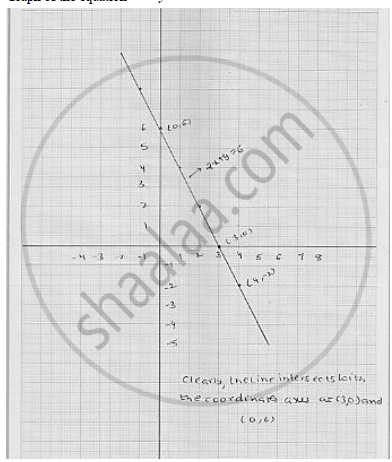# Draw the Graph of the Equation Given Below. Also, Find the Coordinates of the Point Where the Graph Cuts the Coordinate Axes : 2x + Y = 6 - Mathematics

Draw the graph of the equation given below. Also, find the coordinates of the point
where the graph cuts the coordinate axes :  2x + y = 6

#### Solution

we have

2x + y = 6

⇒  y = 6 - 2x      ...........   (1)

Putting  x = 3 in (1) , we get  y = 6 = 2 × 3 = 0

Putting x = 4 in (1) . we get  y = 6 - 2 × 4 = - 2

Thus, we obtain the following table giving coordinates of two points on the line
represented by the equation   2x + y = 6

Graph of the equation  2x + y = 6Concept: Graph of a Linear Equation in Two Variables
Is there an error in this question or solution?

#### APPEARS IN

RD Sharma Mathematics for Class 9
Chapter 7 Linear Equations in Two Variables
Exercise 7.3 | Q 12.3

Share